x

# Drafting Workshop in Discrete Mathematics and Probability 2023

02.06.2023. - 02.08.2023.
Erdős Center

### Description

Several postdoc positions are available in Budapest in various research groups in discrete mathematics and probability theory. The research groups are listed below.

To make the application process more efficient and joyful, we are organizing a drafting workshop for young researchers interested in these positions, with the dates February 6-8, 2023, at the Renyi Institute.

The participants of the workshop can present their work in a research talk, learn more about the research environment in Budapest, meet the heads and members of the research groups in person and in case of a perfect matching, get an offer on the spot.

The application due date is December 18th. The workshop is invitation based. We can cover the accommodation of successful applicants and there is limited funding for travel expenses. If you need travel funding, let us know in your application.

Applications should be sent to the e-mail address large.networks@renyi.hu. Please include 'Drafting workshop application' in the subject of the email. In case the applicant is still a PhD student, the e-mail should contain the expected termination date of their PhD studies.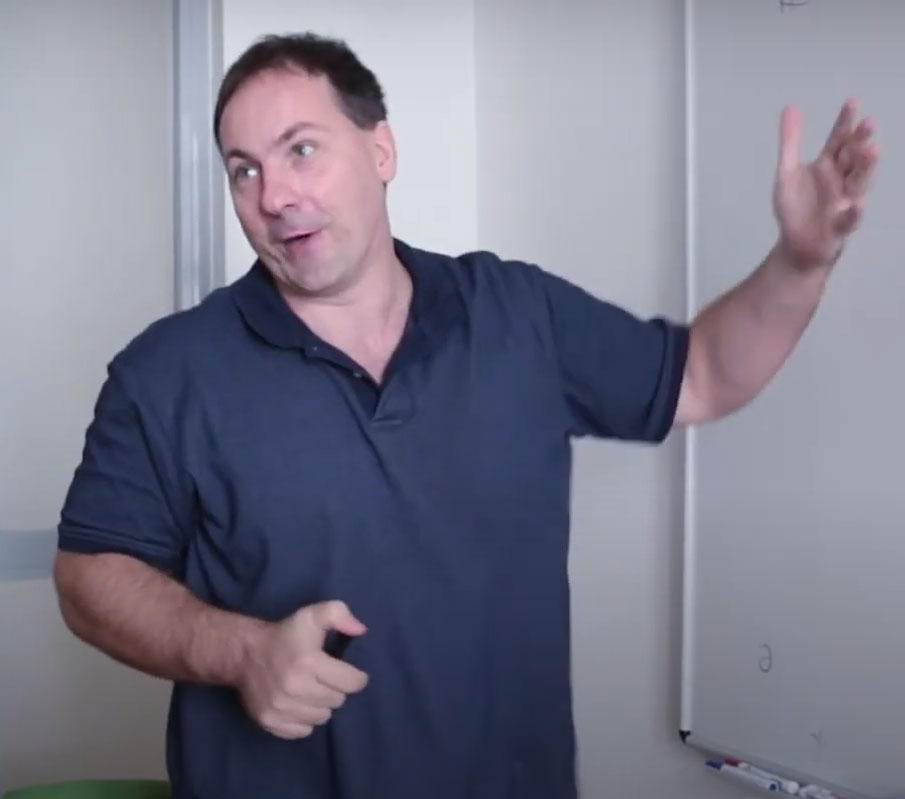Groups and Graph Convergence Research Group

Head: Miklós Abért, Alfréd Rényi Institute of Mathematics

The research group studies measured and asymptotic group theory, in particular spectral theory of graphs and groups, Benjamini-Schramm convergence, stochastic processes on groups, rank gradient, invariant random subgroups, homology growth, sofic entropy and asymptotic invariants of random graphs and locally symmetric spaces.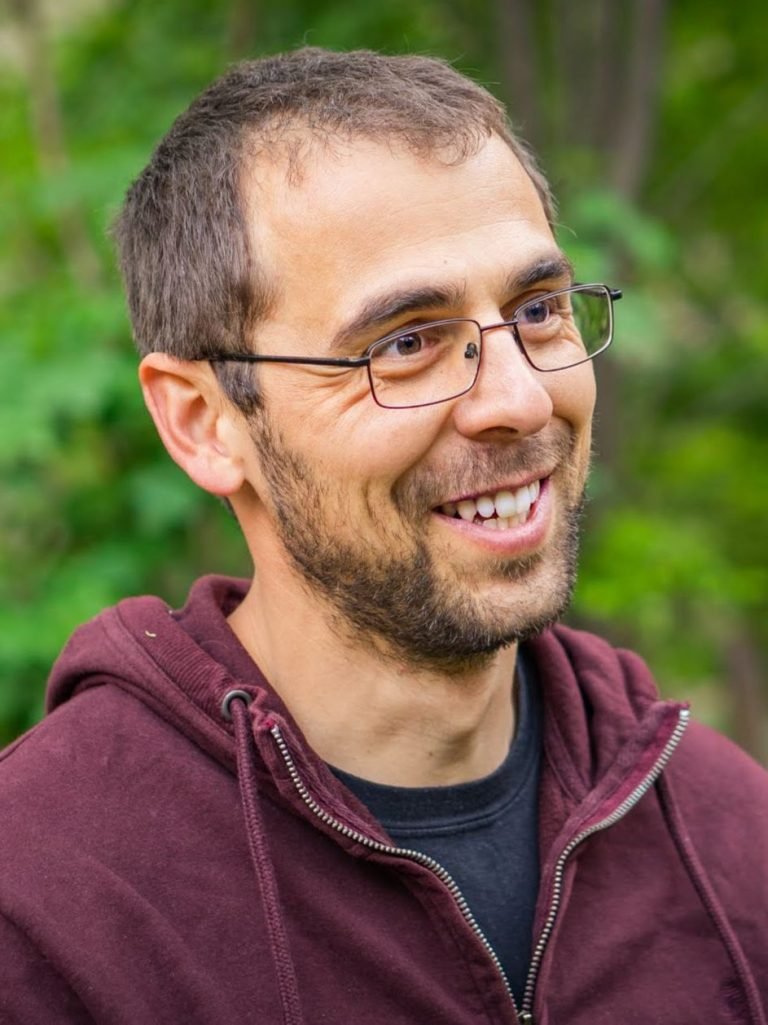Matroid Optimization Research Group (Eötvös Loránd University)

Head: Kristóf Bérczi, Eötvös Loránd University

We aim to explore the global structure of bases in matroids, or more generally, of common bases in the intersection of matroids. Our goal is to devise structural and algorithmic results that will open up new domains for packing common bases algorithmically beyond the currently known special cases. Meanwhile, supported by the results obtained, we aim to resolve a wide list of fundamental open questions that all stem from the global relations of matroid bases.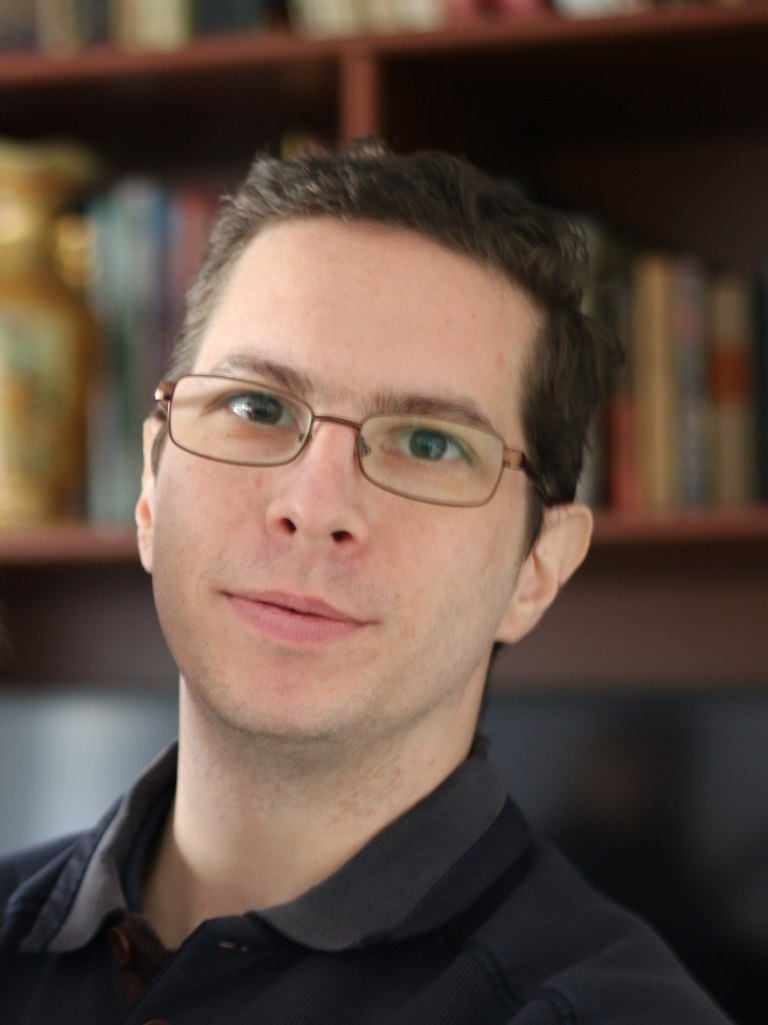Counting in Sparse Graphs Research Group

Head: Péter Csikvári, Alfréd Rényi Institute of Mathematics

We aim to study approximate counting problems of combinatorial objects of a given graph besides restricted information. This general framework includes counting matchings, independent sets, colorings given the degree sequence or local statistics or may be the whole graph as an input. There is an emphasis on developing random and deterministic algorithms.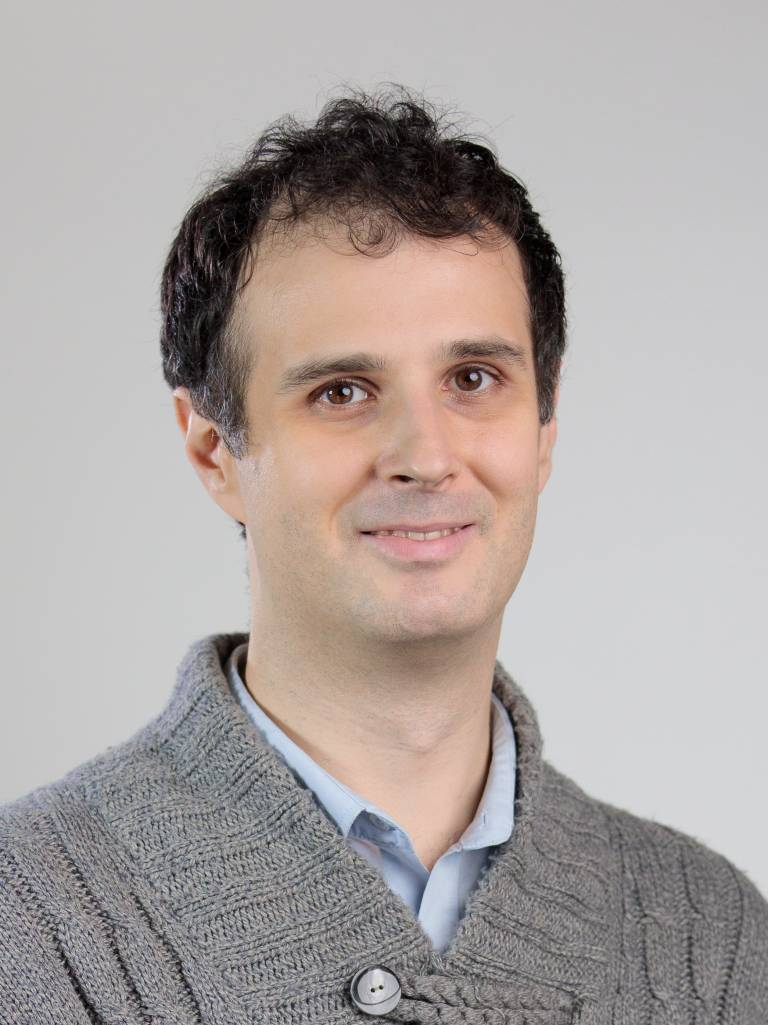Local Algorithms on Sparse Random Graphs Research Group

Head: Endre Csóka, Alfréd Rényi Institute of Mathematics

We examine the structures and phase transitions in large random graphs. For example, the structure of a largest independent set in a random $$d$$-regular graph on $$n → ∞$$ vertices is in a "solid state" for $$d ≥ 20$$ but probably in a "spin glass state" for $$d ≤ 19$$ . We are using the tools of graph limit theory, local algorithms (IID-factor processes), and probability theory, and we are trying to translate and extend some intuitive arguments in statistical physics into this mathematical language.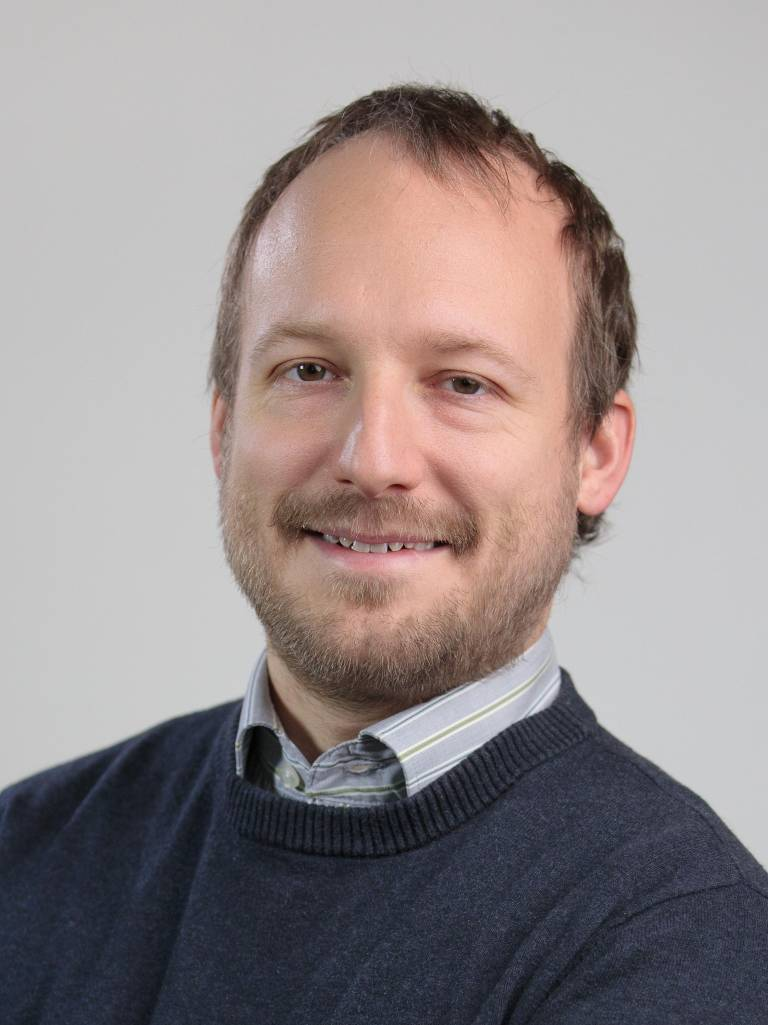Analysis and Applications of Markov Chains Research Group

Head: Balázs Gerencsér, Alfréd Rényi Institute of Mathematics

Our group is analyzing and exploring favorable perturbations of Markov chain transition probabilities towards nding the optimally mixing instance, also investigating minor changes in the transition topology (mostly discrete time chains). Similar research is carried out on a parallel track with an applied mind, in particular for consensus on networked systems, taking into account possibly asynchronous communication and similar challenges.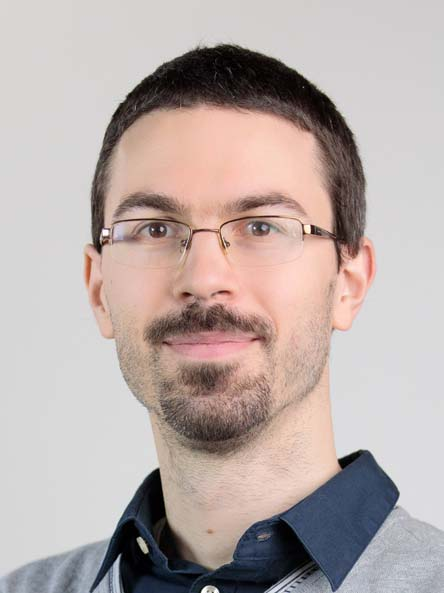Dynamics and Structure of Networks Research Group

Project Leader: Dávid Kunszenti-Kovács, Alfréd Rényi Institute of Mathematics

It is known that the usual integral formula for graph homomorphism densities remains a valid definition for  $$L^p$$ graphons provided the test-graph has degrees bounded by $$p$$, whereas a purely spectral approach connects Schatten norms of compact operators to densities of even cycles. We aim to use tools from functional analysis and probability theory to explore the boundaries of such generalisations of homomorphism densities to singular objects. We are also interested in Inverse Counting Lemma-type results for unbounded graphons, as well as appropriate "Counting Lemmas" for densities in operators.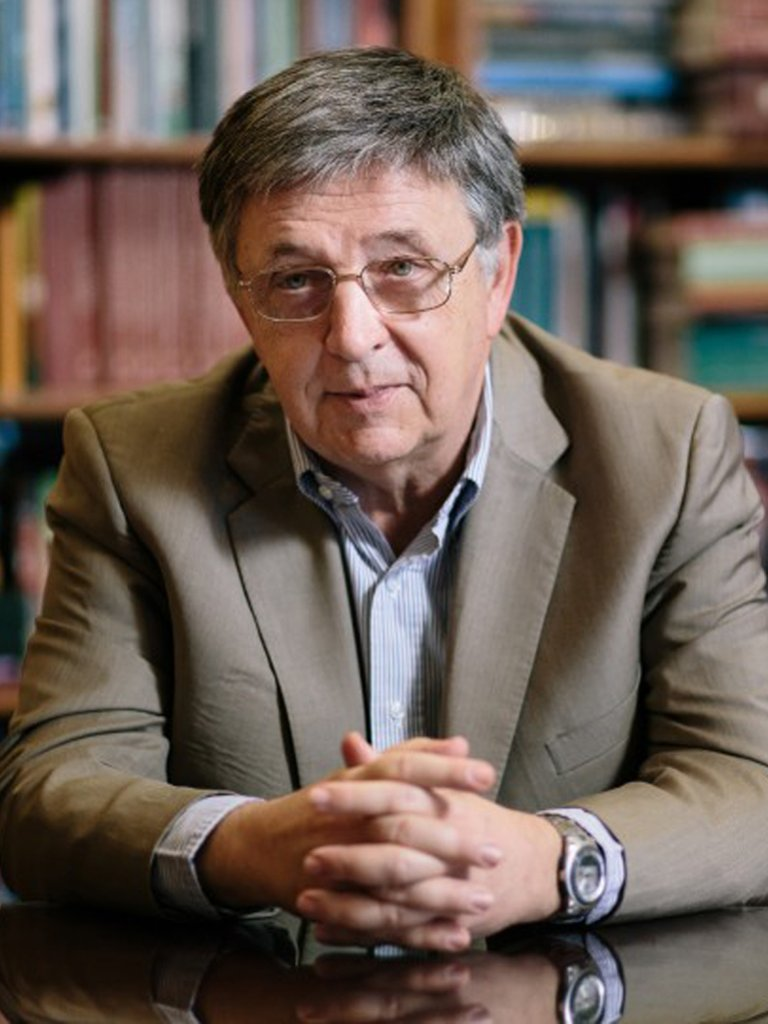Dynamics and Structure of Networks Research Group

Head: László Lovász, Alfréd Rényi Institute of Mathematics

A lot is known about the structure of large networks (graphs), but most real-life networks are interesting because they support interesting dynamics. Our research group is working on extending the structure theory of large networks to the case of graphs with medium density and to graphs supporting dynamics. Motivation comes from graph limit theory, epidemic propagation, and networks embedded in physical space.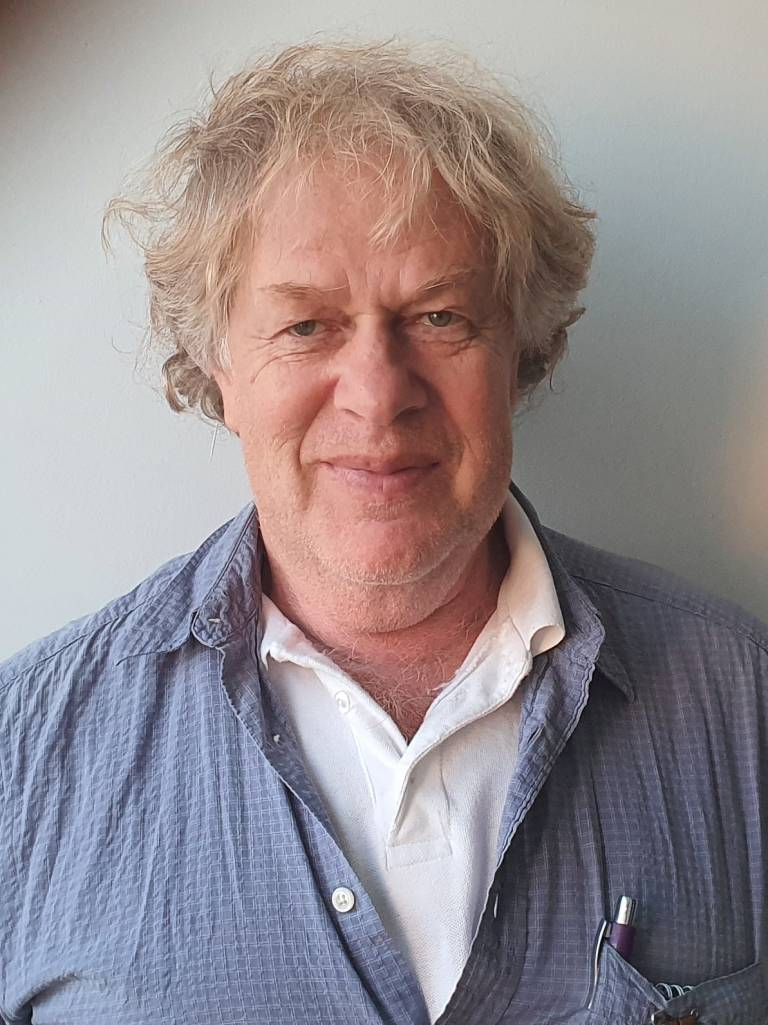GeoScape: Combinatorial Geometry Research Group

Head: János Pach, Alfréd Rényi Institute of Mathematics

A classic theme of combinatorics is to search for interesting patterns in various graphs and hypergraphs. Much of the research in Ramsey-theory, Turán-type extremal graph theory, Szemerédi regularity theory found important applications in combinatorial and computational geometry. However, if we blindly apply these theories in geometric scenarios, the obtained results are rarely optimal. We try to explain this curious phenomenon by proving stronger results on structures often arising in geometric applications: graphs and hypergraphs of bounded complexity, containing no "forbidden" subpattern of a fixed type, or embedded in a surface.

We will focus on some of these problems during the Special Semester on Discrete Geometry and Convexity we run at the Erdős Center of Rényi Institute, August-December 2023.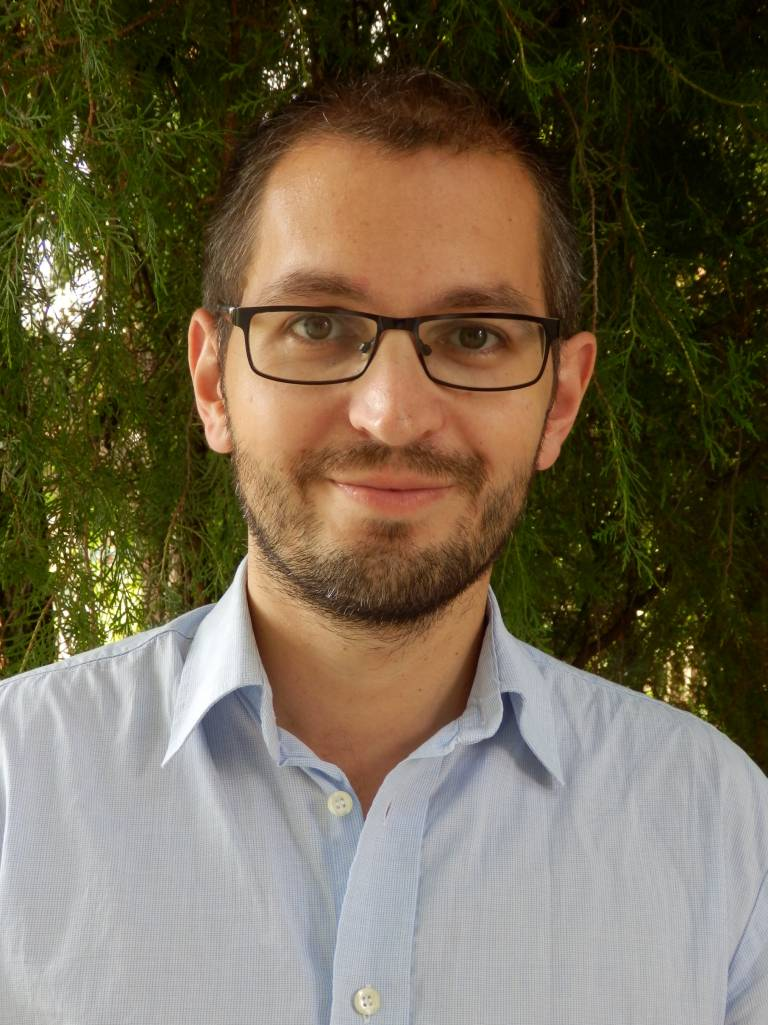Arithmetic Combinatorics Research Group (Budapest University of Technology and Economics)

Head: Péter Pál Pach, Budapest University of Technology and Economics

Our aim is to introduce novel methods to solve problems of various types from Arithmetic Combinatorics. We particularly concentrate on developing techniques built on (linear) algebraic grounds like the slice rank method. A central question to be investigated is the following: What is the largest possible size of a k-AP-free subset of $$\mathbb{Z}_{p}^{n}$$ ?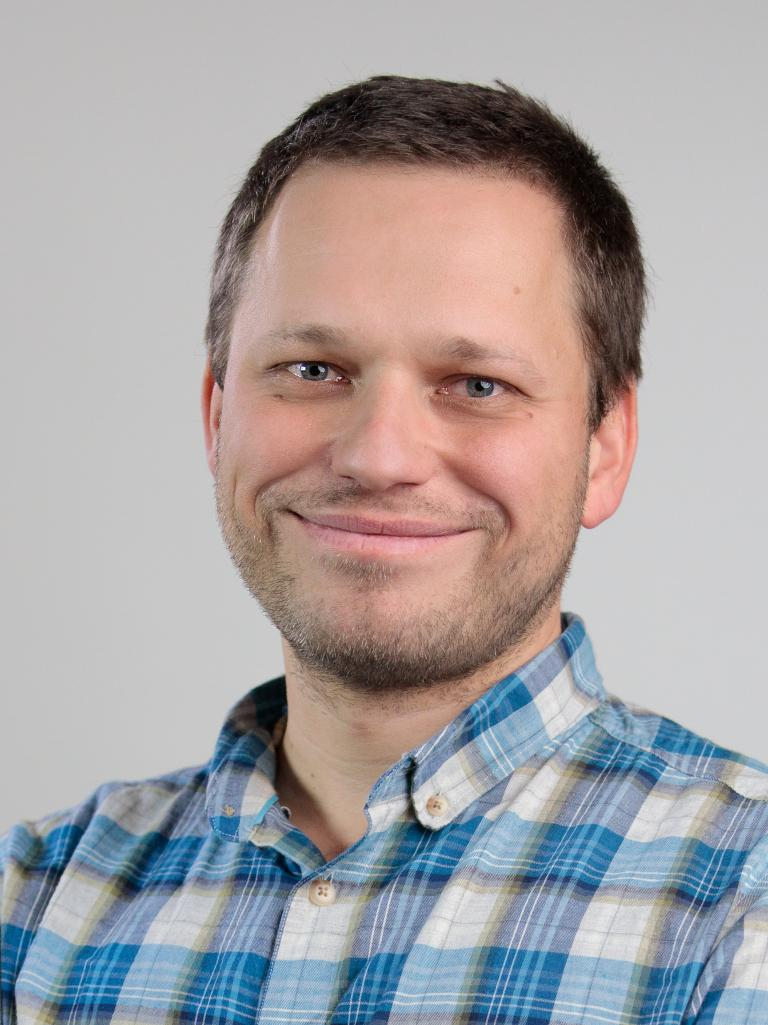Noise-Sensitivity Everywhere Research Group

Head: Gábor Pete, Alfréd Rényi Institute of Mathematics and Budapest University of Technology and Economics

Noise-sensitivity of a Boolean function with random input bits means that resampling a tiny proportion of the input makes the output decorrelate faster than the relaxation time of the entire system. For iid random input, discrete Fourier analysis yields a useful theory to understand what functions are noise-sensitive, with great applications, e.g., in percolation theory. What happens for non-iid input, such as the Ising model, Gaussian random fields, spanning forests, or factor of iid processes? A related goal is to study the (near-)critical behavior of statistical physics models on Cayley graphs of groups and on random graphs.Dynamics and Structure of Networks Research Group

Project Leader: Balázs Ráth, Alfréd Rényi Institute of Mathematics and Budapest University of Technology and Economics

We study random spatial processes and random graph models. These models are designed to capture some real-life phenomena and our goal is to study these phenomena with mathematical rigor. Some of the specific models that we work on include forest fires and frozen percolation, various correlated percolation and epidemic models.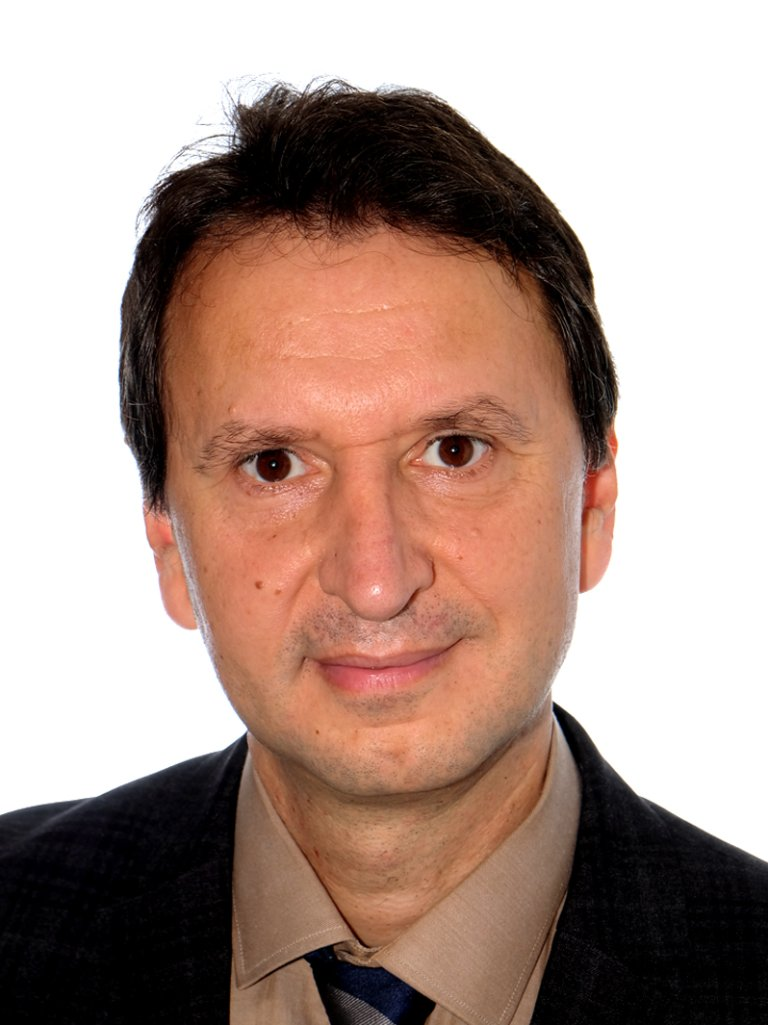Limits of Discrete Structures Research Group

Head: Balázs Szegedy, Alfréd Rényi Institute of Mathematics

We study large networks using methods from analysis, probability theory and algebra. More generally we develop limit concepts for various discrete structures.
Other closely related research areas include higher order Fourier analysis, dynamical systems and even machine learning.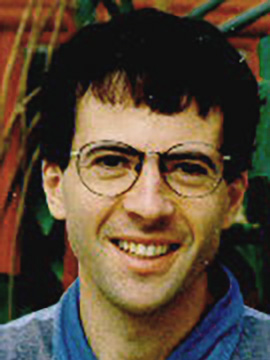Effective Random Methods in Discrete Mathematics Reasearch Group

Head: Gábor Tardos, Alfréd Rényi Institute of Mathematics

The probabilistic method, pioneered by Paul Erdős, can show the existence of combinatorial objects without hinting how to construct them effectively. Recent developments concerning the constructive version of Lovász Local Lemma (LLL) showed how to modify the probabilistic method to make it effective. We plan to concentrate on applications in combinatorics, measure theory, geometry, and cryptography.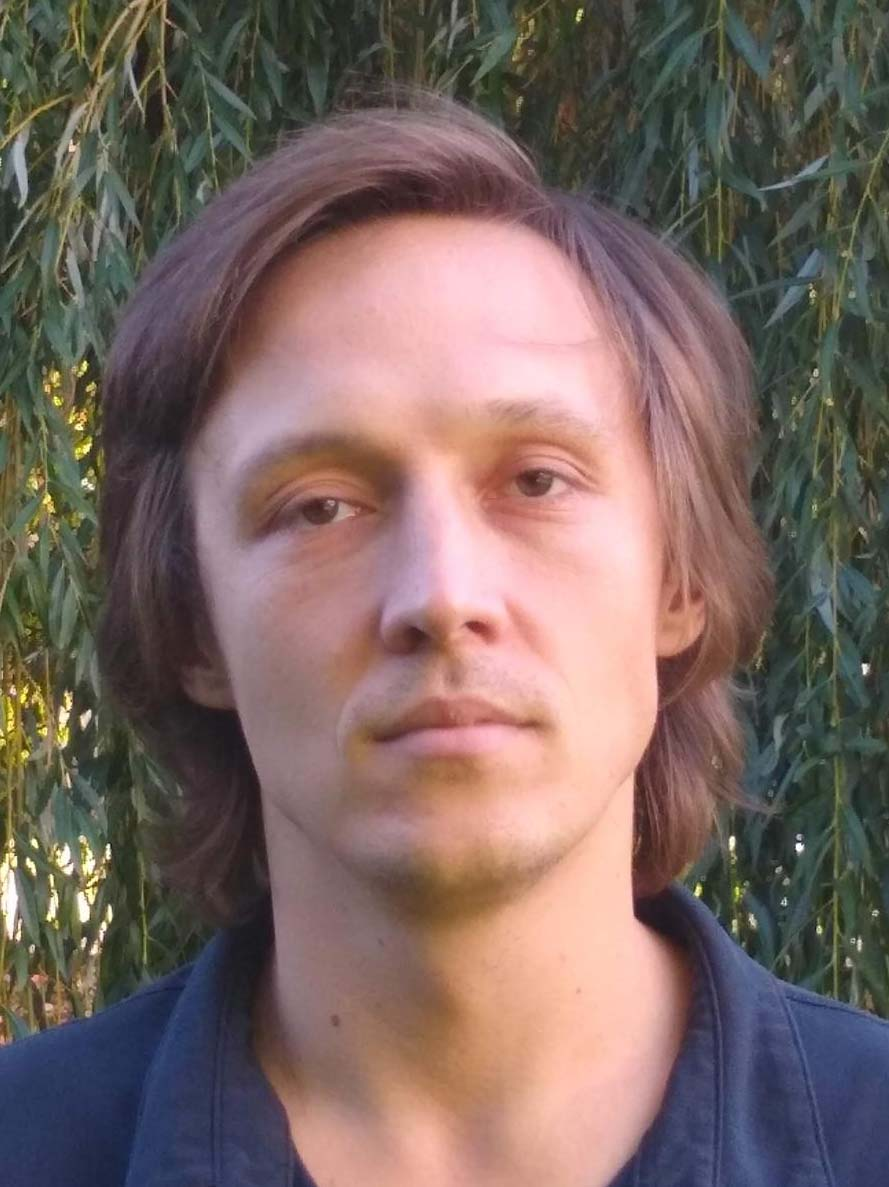Borel Combinatorics and Complexity Research Group (Eötvös Loránd University)

Head: Zoltán Vidnyánszky, Eötvös Loránd University

Our research group investigates infinite graphs and their combinatorial properties, but, instead of the measurability requirement typically present in the case of graphons/graphings, we consider definable (e.g., Borel) graphs and chromatic numbers. It turns out that there are numerous connections of these objects to computational complexity and distributed computing.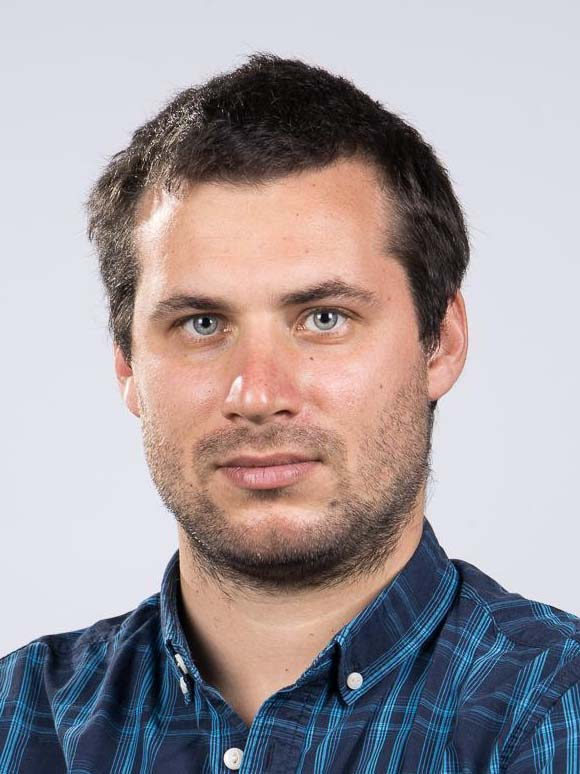Optimal Transport Research Group

Head: Dániel Virosztek, Alfréd Rényi Institute of Mathematics

In the last decades, optimal transport became one of the central topics of analysis with intimate links to mathematical physics, PDE theory, metric geometry, and probability. The theory of quantum optimal transport has been emerging very recently. We study the geometry of classical and quantum structures endowed with transport-related metrics (like the Wasserstein metric) or entropic distances. The main research objectives include the development of quantum optimal transport for general transport costs, the description of the isometry groups of classical and quantum Wasserstein spaces, and the geometric study (with a focus on Riemannian metric tensor, geodesics, and barycenters) of quantum state spaces endowed with entropic dissimilarity measures.

### Organizers

Miklós Abért
Kristóf Bérczi
Péter Csikvári
Endre Csóka
Balázs Gerencsér
Dávid Kunszenti-Kovács
László Lovász
János Pach
Péter Pál Pach
Gábor Pete
Balázs Ráth
Balázs Szegedy
Gábor Tardos
Zoltán Vidnyánszky
Dániel Virosztek